Properties of LTI System

# Properties of LTI System - Notes | Study Signals and Systems - Electrical Engineering (EE)

 1 Crore+ students have signed up on EduRev. Have you?

Properties of LTI System

In the preceding chapters,we have already derived expressions for discrete as well as continuous time convolution operations.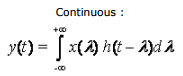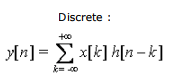We shall now discuss the important properties of convolution for LTI systems.

1) Commutative property : By the commutative property,the following equations hold true :

a) Discrete time: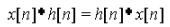Proof : We know that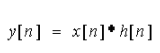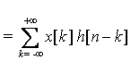Hence we make the following substitution (n - k = l )

∴The above expression can be written as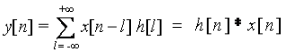So it is clear from the derived expression that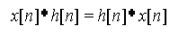Note :

1. 'n' remains constant during the convolution operation so 'n' remains constant in the substitution “n-k = l” even as 'k' and 'l' change.
2. “l” goes from  - ∞ to + ∞  , this would not have been so had 'k' been bounded.( e.g :- 0 < k < 11 would make n < l < n – 11)

b) Continuous Time: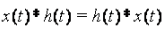Proof:

We Know That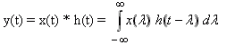Making The Substitution t - λ = Ø               Limits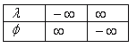dt  = - d λ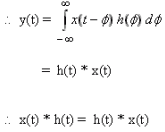Thus we proved that convolution is commutative in both discrete and continuous variables.

Thus the following two systems : One with input signal x(t)and impulse response h(t) and the other with input signal h(t) and impulse response x(t) both give the same output y(t)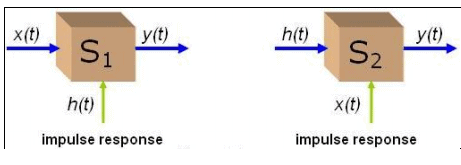2) Distributive Property :

By this property we mean that convolution isdistributive over addition.

a) Discrete :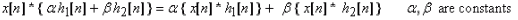b) Continuous :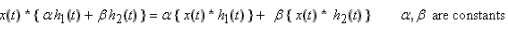A parallel combination of LTI systems can be replaced by an equivalent LTI system which is described by the sum of the individual impulse responses in the parallel combination.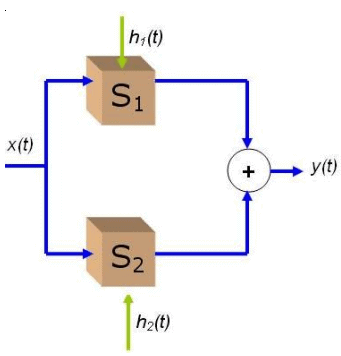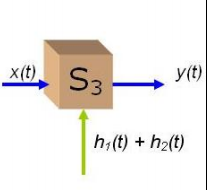3) Associative property

a) Discrete time :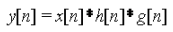Proof : We know that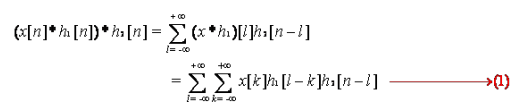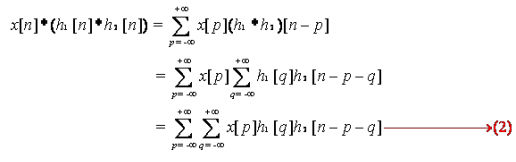Making the substitutions: p = k ; q = (l - k) and comparing the two equations makes our proof complete.
Note: As k and l go -∞ from to +∞  independently of each other, so do p and q, however p depends on k, and q depends on l and k.

b) Continuous time :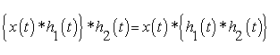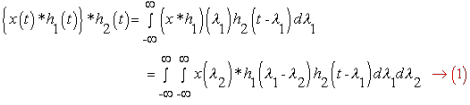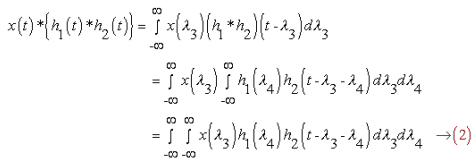Lets substitute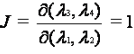The Jacobian for the above transformation is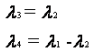Doing some further algebra helps us see equation (2) transforming into equation (1) ,i.e. essentially they are the same. The limits are also the same. Thus the proof is complete.

Implications

This property (Associativity) makes the representation y[n] = x[n]*h[n] *g[n] unambiguous. From this property, we can conclude that the effective impulse response of acascaded LTI system is given by the convolution of their individual impulse responses.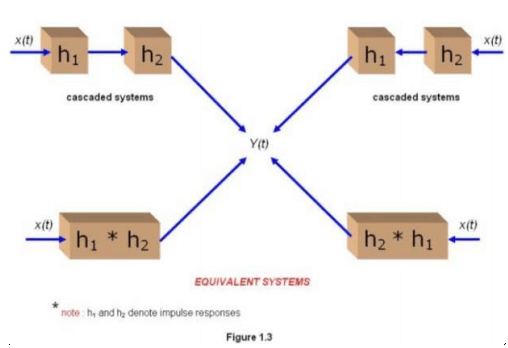Consequently the unit impulse response of a cascaded LTI system is independent of the order in which the individual LTI systems are connected. Note :All the above three properties are certainly obeyed by LTI systemsbuthold for non-LTI systems in, as seen from the following example: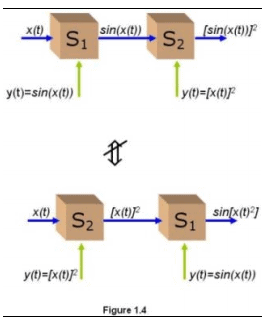4) LTI systems and Memory
Recall that a system is memoryless if its output depends on the current input only. From the expression :

It is easily seen that y[n] depends only on x[n] if and only if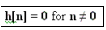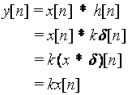5) Invertibility :

A system is said to be invertible if there exists an inverse system which when connected in series with the original system produces an output identical to the input.

We know that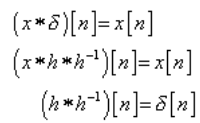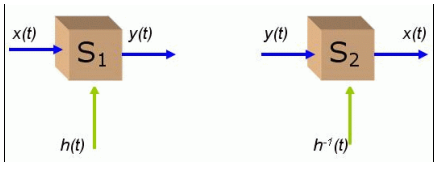6) Causality :

a) Discrete time :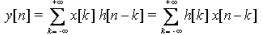In order for a discrete LTI system to be causal, y[n] must not depend on x[k] for k > n. For this to be true h[n-k]'s corresponding to the x[k]'s for k > n must be zero. This then requires the impulse response of a causal discrete time LTI system satisfy the following conditions :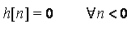Essentially the system output depends only on the past and the present values of the input.

Proof : ( By contradiction )

Let in particular h[k] is not equal to 0, for some k<0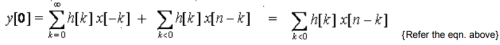So we need to prove that for all x[n] = 0, n < 0, y = 0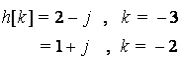Now we take a signal defined as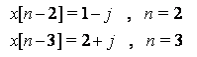This signal is zero elsewhere. Therefore we get the following result :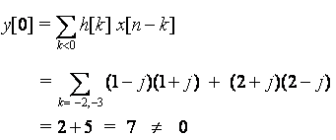We have come to the result that y ≠ 0, for the above assumption. ∴ our assumption stands void. So we conclude that y[n] cannot be independent of x[k] unless h[k] = 0 for k < 0

Note : Here we ensured a non-zero summation by choosing x[n-k]'s as conjugate of h[k]'s.

b) Continuous time :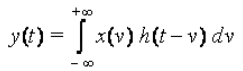In order for a continuous LTI system to be causal, y(t) must not depend on x(v) for v > t . For this to be true h(t-v)’s corresponding to the x(v)’s for v > t must be zero. This then requires the impulse response of a causal continuous time LTI system satisfy the following conditions :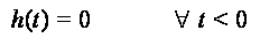As stated before in the discrete time analysis,the system output depends only on the past and the present values of the input.
Proof : ( By contradiction )

Suppose, there exists a > 0 such that h(-a)≠0

Now consider  x(t) = δ(t-α)

Since,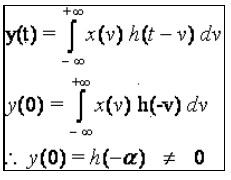• System is not causal, a contradiction. Hence,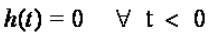7) Stability :

A system is said to be stable if its impulse response satisfies the following criterion :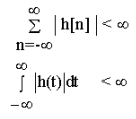Theorem:

Stability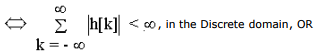Stability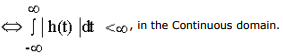Proof of sufficiency:

Suppose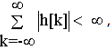We have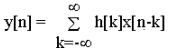If x[n] is bounded i.e.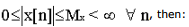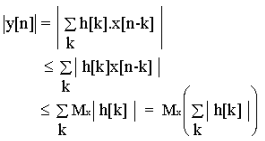But as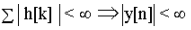Proof of Necessity:

Take any n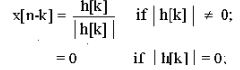If | h[k] | = 0, then x[n-k] is bounded with bound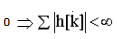Then,Hence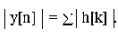But since the system is stable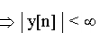which in turn implies that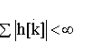. Hence if y[n] is bounded then the conditionmust hold.

Hence Proved A similar proof follows in continuous time when you replace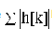by integral .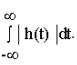The document Properties of LTI System - Notes | Study Signals and Systems - Electrical Engineering (EE) is a part of the Electrical Engineering (EE) Course Signals and Systems.
All you need of Electrical Engineering (EE) at this link: Electrical Engineering (EE)

## Signals and Systems

40 videos|44 docs|33 tests
 Use Code STAYHOME200 and get INR 200 additional OFF

## Signals and Systems

40 videos|44 docs|33 tests

Track your progress, build streaks, highlight & save important lessons and more!

,

,

,

,

,

,

,

,

,

,

,

,

,

,

,

,

,

,

,

,

,

;